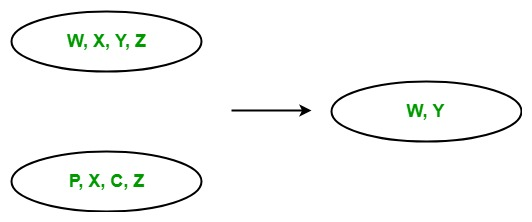# LINQ | Set Operator | Except

• Last Updated : 22 May, 2019

In LINQ, Set operators are those operators in query expression which return a result set based on the existence or non-existence of the equivalent elements within the same or different collections or sequences or sets. The standard query operator contains the following set operators:

1. Union
2. Intersect
3. Except
4. Distinct

#### Except Operator

The Except operator returns the set difference. Or in other words, we can say that it returns the set or collection which contain the elements that do not appear in the second collection or set.• It does not support Query Syntax in C# and VB.Net languages. But you can use Except method on query variable or you can wrap your query in brackets and then call Except method.
• It support method syntax in both C# and VB.Net languages.
• It present in both the Queryable and Enumerable class.
• It is implemented by using deferred execution.
• When you are working with the collections of complex types, then you must use IEqualityComparer interface, otherwise, the Except method will give you incorrect result.

Example 1:

 `// C# program to find the difference``// of the given sequences``using` `System;``using` `System.Linq;`` ` `class` `GFG {`` ` `    ``static` `public` `void` `Main()``    ``{`` ` `        ``// Data source``        ``char``[] sequence1 = {``'m'``, ``'q'``, ``'o'``, ``'s'``, ``'y'``, ``'a'``};``        ``char``[] sequence2 = {``'p'``, ``'t'``, ``'r'``, ``'s'``, ``'y'``, ``'z'``};`` ` `        ``// Display the sequences``        ``Console.WriteLine(``"Sequence 1 is: "``);`` ` `        ``foreach``(``var` `s1 ``in` `sequence1)``        ``{``            ``Console.WriteLine(s1);``        ``}`` ` `        ``Console.WriteLine(``"Sequence 2 is: "``);`` ` `        ``foreach``(``var` `s2 ``in` `sequence2)``        ``{``            ``Console.WriteLine(s2);``        ``}`` ` `        ``// Get the difference of the given ``        ``// sequences Using Except function``        ``var` `result = sequence1.Except(sequence2);`` ` `        ``Console.WriteLine(``"New Sequence: "``);`` ` `        ``foreach``(``var` `val ``in` `result)``        ``{``            ``Console.WriteLine(val);``        ``}``    ``}``}`
Output:
```Sequence 1 is:
m
q
o
s
y
a
Sequence 2 is:
p
t
r
s
y
z
New Sequence:
m
q
o
a
```

Example 2:

 `// C# program to find the languages which is ``// not known by employees of the Department 2``using` `System;``using` `System.Linq;``using` `System.Collections.Generic;`` ` `// Employee details``// Department 1``public` `class` `Employee1 {`` ` `    ``public` `int` `emp_id1``    ``{``        ``get``;``        ``set``;``    ``}`` ` `    ``public` `string` `emp_name1``    ``{``        ``get``;``        ``set``;``    ``}``    ``public` `string` `emp_lang1``    ``{``        ``get``;``        ``set``;``    ``}``}`` ` `// Employee details``// Department 2``public` `class` `Employee2 {`` ` `    ``public` `int` `emp_id2``    ``{``        ``get``;``        ``set``;``    ``}`` ` `    ``public` `string` `emp_name2``    ``{``        ``get``;``        ``set``;``    ``}``    ``public` `string` `emp_lang2``    ``{``        ``get``;``        ``set``;``    ``}``}`` ` `public` `class` `GFG {`` ` `    ``// Main method``    ``static` `public` `void` `Main()``    ``{``        ``List emp1 = ``new` `List() {`` ` `            ``new` `Employee1() {emp_id1 = 209, emp_name1 = ``"Anjita"``,``                                               ``emp_lang1 = ``"C#"``},`` ` `            ``new` `Employee1() {emp_id1 = 210, emp_name1 = ``"Soniya"``,``                                                ``emp_lang1 = ``"C"``},`` ` `            ``new` `Employee1() {emp_id1 = 211, emp_name1 = ``"Rohit"``,``                                            ``emp_lang1 = ``"Java"``},`` ` `        ``};`` ` `        ``List emp2 = ``new` `List() {`` ` `            ``new` `Employee2() {emp_id2 = 290, emp_name2 = ``"Anjita"``,``                                               ``emp_lang2 = ``"C#"``},`` ` `            ``new` `Employee2() {emp_id2 = 212, emp_name2 = ``"MaMa"``,``                                         ``emp_lang2 = ``"Python"``},`` ` `            ``new` `Employee2() {emp_id2 = 233, emp_name2 = ``"Rima"``,``                                           ``emp_lang2 = ``"Java"``},`` ` `        ``};`` ` `        ``// Query to find the languages that is not``        ``// known by employees of the department 2``        ``// Using Except method``        ``var` `res = emp1.Select(e => e.emp_lang1).Except(emp2.Select(e => e.emp_lang2));``         ` `        ``Console.WriteLine(``"Language: "``);``         ` `        ``foreach``(``var` `val ``in` `res)``        ``{``            ``Console.WriteLine(val);``        ``}``    ``}``}`
Output:
```Language:
C
```

My Personal Notes arrow_drop_up